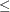## Numerical Difficulties

Extremely large or extremely small numerical values might cause computational difficulties (singularities, stalled solution progress, false infeasibilities, and so on) for optimization solvers, but the occurrence of such difficulties is hard to predict. For this reason, solvers issue a data error message when they detect model data that exceed a specific threshold number. The value of the threshold number depends on your operating environment and is printed in the log as part of the data error message.

The following conditions produce a data error:

• The absolute value of an objective coefficient, constraint coefficient, or range (difference between the upper and lower bounds on a constraint) is greater than the threshold number.

• A variable’s lower bound, aorconstraint’s right-hand side, or a range constraint’s lower bound is greater than the threshold number.

• A variable’s upper bound, aorconstraint’s right-hand side, or a range constraint’s upper bound is smaller than the negative threshold number.

If a variable’s upper bound is greater than 1E20, then solvers treats the bound as. Similarly, if a variable’s lower bound is less than –1E20, then LP solver treats the bound as.

If a solver fails or experiences numerical difficulties when solving a problem, try one of the following remedies:

• Improve the input data: Rescale very large and very small numbers in constraints, objectives, right-hand sides, and variable bounds. It is recommended that the magnitudes of the largest and smallest constraint coefficients not exceed 1E6.

• Specify different algorithms or options (or both): For example, to solve a linear program, you can choose from the primal simplex, dual simplex, interior point, and network simplex algorithms. Using available options, you can tighten or relax feasibility or optimality tolerances.